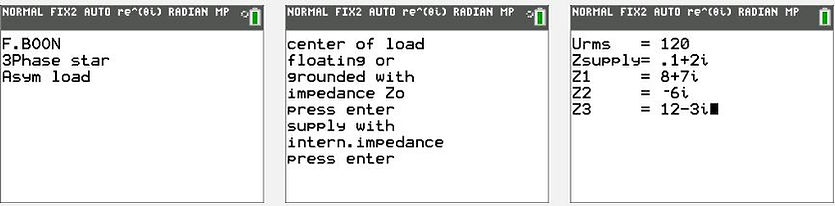naver-site-verification: naver47238a1b6bfbb19a2fd4b619734fa9a6.html
top of page

# Three-Phase asymmetric load calculation program using                          the Ti-84 plus (CE)

## Calculate power, power factor, reactive power, current etc. for each phase

In electrical engineering 3 phase systems are very common. A special Ti-84 program is available to analyze three-phase systems with asymmetric or symmetric load in star configuration. Floating star point  (schematic 1) or grounding it with an impedance Zo (schematic 2) is possible. Additionally, an extra impedance Zsupply for cables or the internal impedance of the source can be included.
Voltages
(V), currents(A) , and powers are shown in complex mode. Mode degree gives the results in degrees.
As the complex power P =UI* = a+ib this is read as follows: Ptrue(W)= a,  Pq(Var) =b.

If you have an asymmetric delta configuration, then use the program delta to star conversion first.
The output of that program (X, Y, Z)  can be used as input in this program by substituting : Z1=X, Z2=Y, Z3=Z and choosing the option floating star?=YES. Remind that in this case, the floating voltage has no physical meaning.
First example :
A three-phase system with floating star, asymmetric load.
Urms is the RMS source voltage of one phase. The phase shifts of the other phase voltages are automatically calculated for a clockwise (normal) system. An inverse anti-clockwise system is explained on the page:
Zsupply (Ohm) is the internal impedance of the source or cable to the load.
Uzsup(1,2,3) (V) = rms voltage loss over Zsupply.
Zsupply = 0 Ω is permitted to be chosen. The difference between Psup and P1,P2,P3 is that in Psup losses of Zsupply are included. Psup is the complex power of a source.### An example of an asymmetric star load with floating star point### Example of asymmetric star load with a grounded star point with an impedance Zo